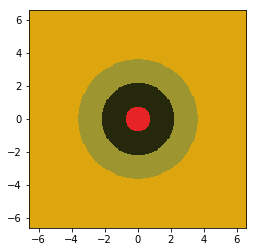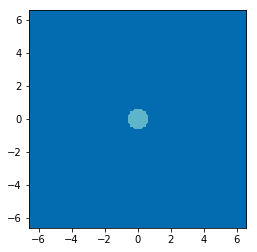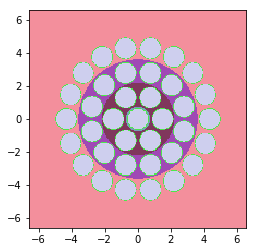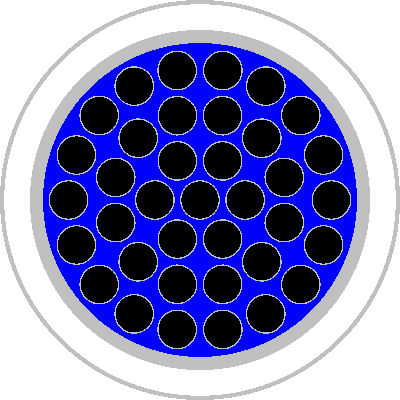# Modeling a CANDU Bundle¶

In this example, we will create a typical CANDU bundle with rings of fuel pins. At present, OpenMC does not have a specialized lattice for this type of fuel arrangement, so we must resort to manual creation of the array of fuel pins.

:

%matplotlib inline
from math import pi, sin, cos
import numpy as np
import openmc


Let’s begin by creating the materials that will be used in our model.

:

fuel = openmc.Material(name='fuel')
fuel.set_density('g/cm3', 10.0)

heavy_water = openmc.Material(name='heavy water')
heavy_water.set_density('g/cm3', 1.1)


With our materials created, we’ll now define key dimensions in our model. These dimensions are taken from the example in section 11.1.3 of the Serpent manual.

:

# Outer radius of fuel and clad
r_fuel = 0.6122

# Pressure tube and calendria radii
pressure_tube_ir = 5.16890
pressure_tube_or = 5.60320
calendria_ir = 6.44780
calendria_or = 6.58750

# Radius to center of each ring of fuel pins
ring_radii = np.array([0.0, 1.4885, 2.8755, 4.3305])


To begin creating the bundle, we’ll first create annular regions completely filled with heavy water and add in the fuel pins later. The radii that we’ve specified above correspond to the center of each ring. We actually need to create cylindrical surfaces at radii that are half-way between the centers.

:

# These are the surfaces that will divide each of the rings
radial_surf = [openmc.ZCylinder(r=r) for r in

water_cells = []
# Create annular region
if i == 0:
elif i == ring_radii.size - 1:
else:

water_cells.append(openmc.Cell(fill=heavy_water, region=water_region))


Let’s see what our geometry looks like so far. In order to plot the geometry, we create a universe that contains the annular water cells and then use the Universe.plot() method. While we’re at it, we’ll set some keyword arguments that can be reused for later plots.

:

plot_args = {'width': (2*calendria_or, 2*calendria_or)}
bundle_universe = openmc.Universe(cells=water_cells)
bundle_universe.plot(**plot_args)

:

<matplotlib.image.AxesImage at 0x154b1fe8f438>Now we need to create a universe that contains a fuel pin. Note that we don’t actually need to put water outside of the cladding in this universe because it will be truncated by a higher universe.

:

surf_fuel = openmc.ZCylinder(r=r_fuel)

fuel_cell = openmc.Cell(fill=fuel, region=-surf_fuel)


:

pin_universe.plot(**plot_args)

:

<matplotlib.image.AxesImage at 0x154b1de334a8>The code below works through each ring to create a cell containing the fuel pin universe. As each fuel pin is created, we modify the region of the water cell to include everything outside the fuel pin.

:

num_pins = [1, 6, 12, 18]
angles = [0, 0, 15, 0]

for i, (r, n, a) in enumerate(zip(ring_radii, num_pins, angles)):
for j in range(n):
# Determine location of center of pin
theta = (a + j/n*360.) * pi/180.
x = r*cos(theta)
y = r*sin(theta)

water_cells[i].region &= +pin_boundary

# Create each fuel pin -- note that we explicitly assign an ID so
# that we can identify the pin later when looking at tallies
pin = openmc.Cell(fill=pin_universe, region=-pin_boundary)
pin.translation = (x, y, 0)
pin.id = (i + 1)*100 + j

:

bundle_universe.plot(**plot_args)

:

<matplotlib.image.AxesImage at 0x154b1ddaadd8>Looking pretty good! Finally, we create cells for the pressure tube and calendria and then put our bundle in the middle of the pressure tube.

:

pt_inner = openmc.ZCylinder(r=pressure_tube_ir)
pt_outer = openmc.ZCylinder(r=pressure_tube_or)
calendria_inner = openmc.ZCylinder(r=calendria_ir)
calendria_outer = openmc.ZCylinder(r=calendria_or, boundary_type='vacuum')

bundle = openmc.Cell(fill=bundle_universe, region=-pt_inner)
pressure_tube = openmc.Cell(fill=clad, region=+pt_inner & -pt_outer)
v1 = openmc.Cell(region=+pt_outer & -calendria_inner)
calendria = openmc.Cell(fill=clad, region=+calendria_inner & -calendria_outer)

root_universe = openmc.Universe(cells=[bundle, pressure_tube, v1, calendria])


Let’s look at the final product. We’ll export our geometry and materials and then use plot_inline() to get a nice-looking plot.

:

geometry = openmc.Geometry(root_universe)
geometry.export_to_xml()

materials = openmc.Materials(geometry.get_all_materials().values())
materials.export_to_xml()

:

plot = openmc.Plot.from_geometry(geometry)
plot.color_by = 'material'
plot.colors = {
fuel: 'black',
heavy_water: 'blue'
}
plot.to_ipython_image()

:## Interpreting Results¶

One of the difficulties of a geometry like this is identifying tally results when there was no lattice involved. To address this, we specifically gave an ID to each fuel pin of the form 100*ring + azimuthal position. Consequently, we can use a distribcell tally and then look at our DataFrame which will show these cell IDs.

:

settings = openmc.Settings()
settings.particles = 1000
settings.batches = 20
settings.inactive = 10
settings.source = openmc.Source(space=openmc.stats.Point())
settings.export_to_xml()

:

fuel_tally = openmc.Tally()
fuel_tally.filters = [openmc.DistribcellFilter(fuel_cell)]
fuel_tally.scores = ['flux']

tallies = openmc.Tallies([fuel_tally])
tallies.export_to_xml()

:

openmc.run(output=False)


The return code of 0 indicates that OpenMC ran successfully. Now let’s load the statepoint into a openmc.StatePoint object and use the Tally.get_pandas_dataframe(...) method to see our results.

:

sp = openmc.StatePoint('statepoint.{}.h5'.format(settings.batches))

:

output_tally = sp.get_tally()
output_tally.get_pandas_dataframe()

:

level 1 level 2 level 3 distribcell nuclide score mean std. dev.
univ cell univ cell univ cell
id id id id id id
0 3 44 1 100 2 5 0 total flux 0.207805 0.007037
1 3 44 1 200 2 5 1 total flux 0.197197 0.005272
2 3 44 1 201 2 5 2 total flux 0.190310 0.007816
3 3 44 1 202 2 5 3 total flux 0.194736 0.006469
4 3 44 1 203 2 5 4 total flux 0.191097 0.006431
5 3 44 1 204 2 5 5 total flux 0.189910 0.004891
6 3 44 1 205 2 5 6 total flux 0.182203 0.003851
7 3 44 1 300 2 5 7 total flux 0.165922 0.005815
8 3 44 1 301 2 5 8 total flux 0.168933 0.008300
9 3 44 1 302 2 5 9 total flux 0.159587 0.003085
10 3 44 1 303 2 5 10 total flux 0.159158 0.005910
11 3 44 1 304 2 5 11 total flux 0.148537 0.005308
12 3 44 1 305 2 5 12 total flux 0.150945 0.006654
13 3 44 1 306 2 5 13 total flux 0.154237 0.003665
14 3 44 1 307 2 5 14 total flux 0.165888 0.004733
15 3 44 1 308 2 5 15 total flux 0.156777 0.006540
16 3 44 1 309 2 5 16 total flux 0.165277 0.005935
17 3 44 1 310 2 5 17 total flux 0.156528 0.005732
18 3 44 1 311 2 5 18 total flux 0.159610 0.004584
19 3 44 1 400 2 5 19 total flux 0.096597 0.004466
20 3 44 1 401 2 5 20 total flux 0.118214 0.005451
21 3 44 1 402 2 5 21 total flux 0.106167 0.004722
22 3 44 1 403 2 5 22 total flux 0.110814 0.004208
23 3 44 1 404 2 5 23 total flux 0.112319 0.005079
24 3 44 1 405 2 5 24 total flux 0.110232 0.004153
25 3 44 1 406 2 5 25 total flux 0.099967 0.005085
26 3 44 1 407 2 5 26 total flux 0.095444 0.003615
27 3 44 1 408 2 5 27 total flux 0.092620 0.003997
28 3 44 1 409 2 5 28 total flux 0.095517 0.004022
29 3 44 1 410 2 5 29 total flux 0.113737 0.009530
30 3 44 1 411 2 5 30 total flux 0.108368 0.007241
31 3 44 1 412 2 5 31 total flux 0.106990 0.005716
32 3 44 1 413 2 5 32 total flux 0.112050 0.005002
33 3 44 1 414 2 5 33 total flux 0.115054 0.006239
34 3 44 1 415 2 5 34 total flux 0.114394 0.004919
35 3 44 1 416 2 5 35 total flux 0.114352 0.005322
36 3 44 1 417 2 5 36 total flux 0.110890 0.005051

We can see that in the ‘level 2’ column, the ‘cell id’ tells us how each row corresponds to a ring and azimuthal position.